Ratio, proportion and percentages

This free course is available to start right now. Review the full course description and key learning outcomes and create an account and enrol if you want a free statement of participation.

Free course

3.1.1 Try some yourself

Activity 17

Express each of the following percentages as fractions:

• (a) 40%

• (b) 8%

• (c) 70%

• (d)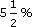• (a) 40%=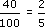• (b) 8%=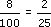• (c) 70%=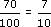• (d)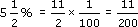Activity 18

Express each of the following percentages as decimals:

• (a) 50%

• (b) 85%

• (c) 7%

• (d)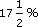• (a) 50% =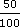= 0.5

• (b) 85% =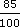=0.85

• (c) 7% =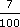= 0.07

• (d)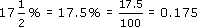Activity 19

A survey was carried out on 840 couples to investigate family income. 75% of the women were in paid work; 90% of the men were in paid work; 18% of the couples had a joint income of less than £160 per week.

• (a) How many women were in paid work?

• (b) How many men were in paid work?

• (c) How many couples had a joint income of less than £160 per week?

• (a) 75% of 840 = 0.75 × 840 = 630,

• so 630 women were in paid work.

• (b) 90% of 840 = 0.9 × 840 = 756,

• so 756 men were in paid work.

• (c) 18% of 840 = 0.18 × 840 = 151.2.

• But 151.2 is not a whole number. So 151 couples had a joint income of less than £160 per week. (The survey result must have been rounded, as a percentage, to the nearest whole number.)

MU120_4M3